# Current date in Hive

Hive provides many date functions to handle the date and time values in the tables. Current_date is one of the date function in hive which returns the current/today’s date. In some cases, we may want to insert the current date for the date column in Hive. To achieve this, We can specify the current_date in the insert query. Similarly we can give the current_date in the select query to get the current date in Hive.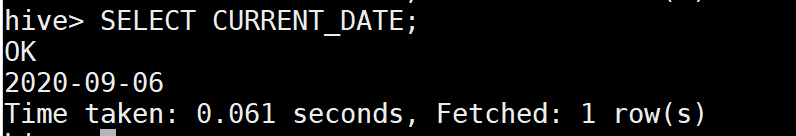Get Current date in Hive

## Add days to Current date in Hive

### Method 1 : DATE_ADD() function

Date_add() function is used to add the days to date value. Lets use this function to add the days with the Current date in Hive.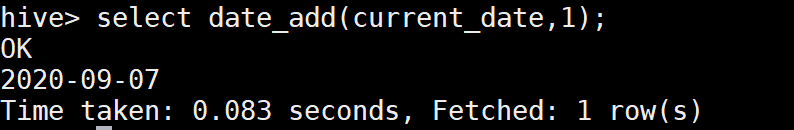date_add function in Hive

### Method 2 : Interval function to add days in Hive

Interval is another date method in Hive which helps to add/subtract the day/month/year from the date value. Here we are going to add the days with the current date value using Interval.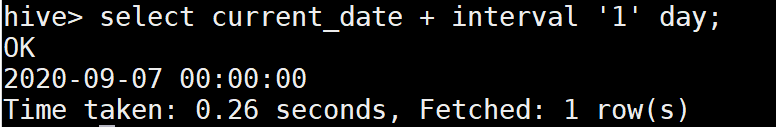Interval function in Hive

As we can see, the Interval function is returning the output in the timestamp format, If we want to change the output vale in Date format (‘YYYY-MM-DD), we can use the date_format() function to handle this in Hive.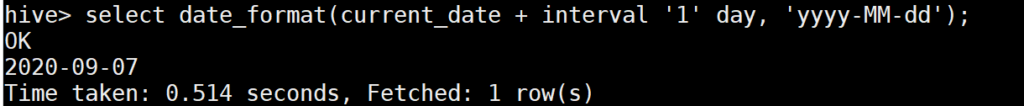Date_format function in Hive

## Subtract days to Current date in Hive

### Method 1 : DATE_SUB() function

Date_Sub() function is used to subtract the number of days from the date value. Lets use this function with the current_date which will provide the yesterday’s date in Hive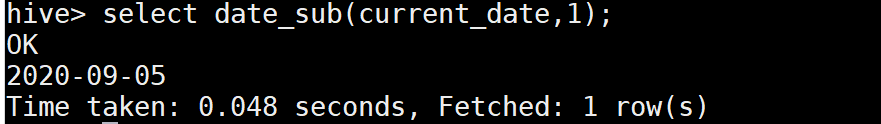DATE_SUB function in Hive

### Method 2 : Interval function to subtract days in Hive

As we know, Interval function handles both add/subtract the days in Hive, we could use this to subtract the days from the Current date also.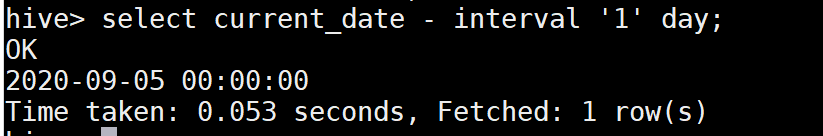Interval to subtract days in Hive